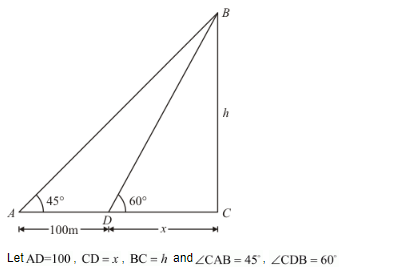# A parachutist is descending vertically and makes angles of elevation of 45° and 60°

Question:

A parachutist is descending vertically and makes angles of elevation of 45° and 60° at two observing points 100 m apart from each other on the left side of himself. Find the maximum height from which he falls and the distance of the point where he falls on the ground form the just observation point.

Solution:

Let BC be the height of the parachutist and makes an angle of elevationsandrespectively at two observing pointsapart from each other.So we use trigonometric ratios.

In triangle BCD

$\tan 60^{\circ}=\frac{h}{x}$

$\Rightarrow x=\frac{h}{\sqrt{3}}$

Now in triangle,

$\tan 45^{\prime}=\frac{h}{x+100}$

$\Rightarrow 1=\frac{h}{x+100}$

$\Rightarrow x+100=h$

$\Rightarrow \frac{h}{\sqrt{3}}+100=h$

$\Rightarrow h+100 \sqrt{3}=\sqrt{3} h$

$\Rightarrow h=\frac{100 \sqrt{3}}{\sqrt{3}-1}$

$\Rightarrow h=50(3+\sqrt{3})$

$\Rightarrow x=\frac{h}{\sqrt{3}}$

$x=\frac{50(3+\sqrt{3})}{\sqrt{3}}$

$=50(1+\sqrt{3})$

Hence the maximum height is $50(3+\sqrt{3}) \mathrm{m}=236.6 \mathrm{~m}$. and distance is $50(1+\sqrt{3}) \mathrm{m}=136.6 \mathrm{~m}$.